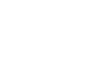What is simplified radical form in math? - letsdiskussVikas joshi

Sales Executive in ICICI Bank | Posted | others

# What is simplified radical form in math?

0
0

digital marketer | Posted

Any perfect squares need to be pulled out from under a square root and left as whole numbers on the outside of, then rationalize the denominator if there is one. Place  “square” with any power “n” now for the general case.

Where n = 1,2,3.........Few examples and there explanation :

a) √︎(72/7) = √︎(36)√︎(2)/√︎(7) = 6√︎(14)/7

b) ³√︎(56/135) = ³√︎(8)³√︎(7)/³√︎(27)³√︎(5) = 2³√︎(7)/3³√︎(5) = 2³√︎(35)/15

c) √︎(64/125) = 2/⁶︎√︎(5³) = 2/√︎(5) = 2√︎(5)/5

For a)   I see 72 is the product of a square and 2 so I pull the square out and leave the √︎(2), I then rationalize the denominator by multiplying by √︎(7)/√︎(7), this leaves 7 in the denominator and results in 14 under the square root in the numerator.

For b) I see both a cube in the numerator and denominator, and I pull them out leaving a non-cube under the cube root in both numerator and denominator, I then rationalize the denominator by multiplying by √︎(5)/√︎(5) and that gives the final answer.

For c) I see a hexic power under the hexic root in the numerator by itself so I simplify to a whole number (2) and a cube (125=5³) under a hexic root in the denominator, I realize that taking ²ⁿ√︎(xⁿ)= √︎(x) and simplify ( √︎(5) ) then I rationalize the denominator by multiplying by √︎(5)/√︎(5).

0
0